• 今天小编就为大家分享一篇matplotlib savefig 保存图片大小的实例，具有很好的参考价值，希望对大家有所帮助。一起跟随小编过来看看吧
• 今天发现sympy依赖的库mpmath里也有很多数学函数，其中也有在复平面绘制二维图的函数cplot，具体例子如下 from mpmath import * def f1(z): return z def f2(z): return z**3 def f3(z): return (z**4-1)**(1/4)...
• ## Matplotlib

万次阅读 多人点赞 2018-08-23 23:28:21
matplotlib.pyplot 是一个命令风格的函数集合，这使得 matplotlib 工作起来和MATLAB很相似。每一个 pyplot 函数都会使图形发生一些变化，例如：创建一幅图形、在一幅图中创建一个绘图区域、在绘图区域中绘制一些线.....
官网帮助文档地址：https://matplotlib.org/tutorials/introductory/pyplot.html
Pyplot简介
matplotlib.pyplot 是一个命令风格的函数集合，这使得 matplotlib 工作起来和MATLAB很相似。每一个 pyplot 函数都会使图形发生一些变化，例如：创建一幅图形、在一幅图中创建一个绘图区域、在绘图区域中绘制一些线、使用标签对图形进行修饰，等等。在matplotlib.pyplot中，各种各样的状态通过调用不同的函数被保存下来，因此，matplotlib 能够持续地对例如：当前的图形和绘图区域、并将绘图函数作用到当前轴上（请注意，这里和文档中大多数地方的“轴”指的是图形的坐标系部分，而不是严格数学术语中的多个轴）。            使用 pyplot 生成一个可视化效果非常快：
import matplotlib.pyplot as plt
plt.plot([1,2,3,4])
plt.ylabel('some numbers')
plt.show()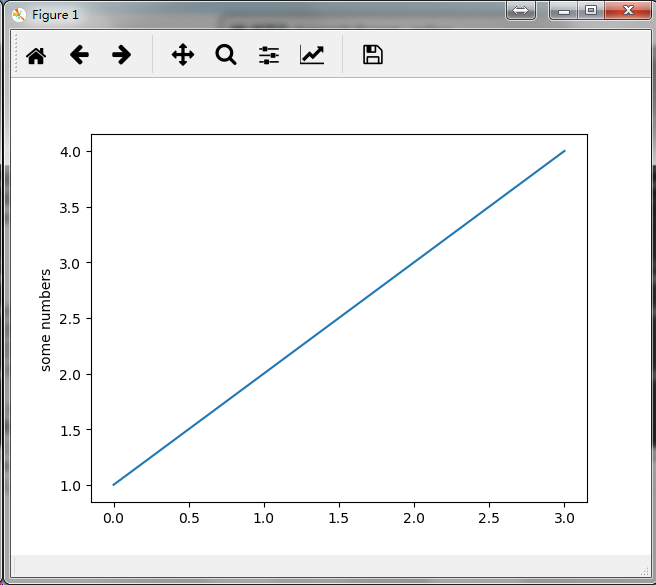你可能会觉得很奇怪：为什么X轴取值的范围是 0~3，而Y轴取值的范围是1~4 ？
这是因为：如果你只提供 了一个列表或者数组作为 plot() 命令的参数，Matplotlib 就会把这个序列当作是Y轴上的取值，并自动地为你生成 X轴上的值。由于在python中范围是从0开始的，因此在默认情况下，X轴向量会与Y轴向量的长度相同但其取值会从0开始，正如我们所看到的X轴的数据是[0,1,2,3]。            plot() 是一个多功能的命令，能够接收任意数量的参数，例如：你可以使用如下命令来绘制与X相对的Y的图形。
plt.plot([1, 2, 3, 4], [1, 4, 9, 16])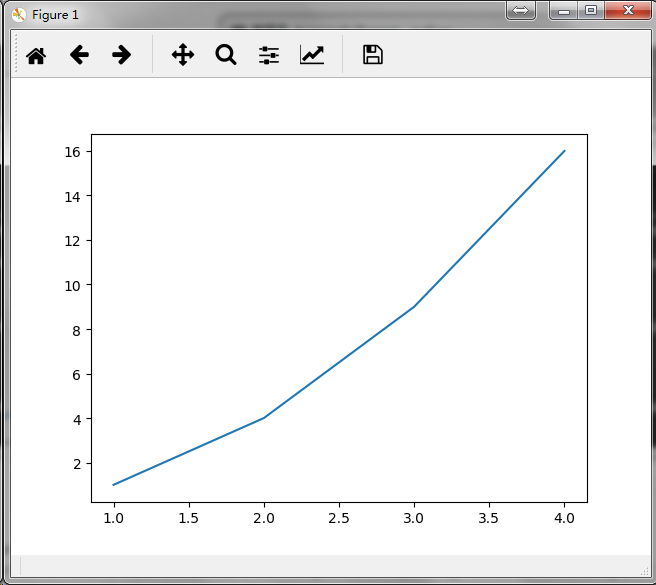格式化图形样式
plot() 还为每一对参数X和Y提供了一个可选的第三个格式化字符串参数用来指定图形的颜色和线型。该格式化字符串的字母和符号均来自于MATLAB，由一个代表颜色的字符串和一个代表线型的字符串组合而成。默认使用的格式化字符串是’b-‘（表示蓝色实线）。             如果想要使用红色的圆点来绘制上例中的图形，你可以这么写：
plt.plot([1, 2, 3, 4], [1, 4, 9, 16], 'ro')
plt.axis([0, 6, 0, 20])
plt.show()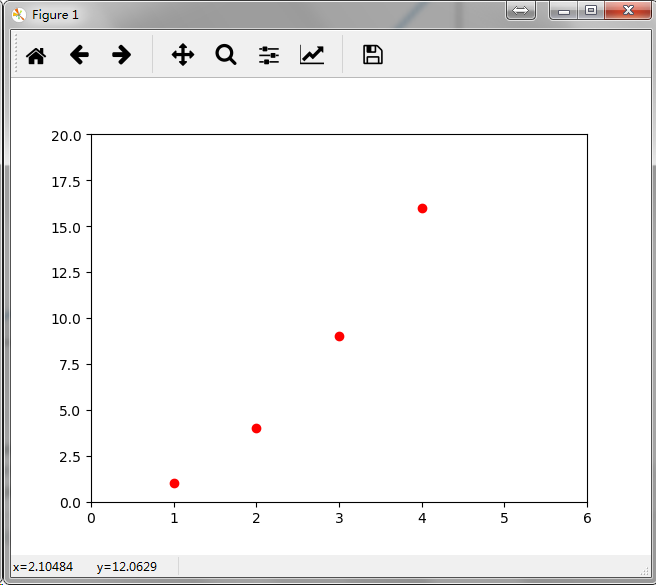你可以查看 plot() 的文档来获取关于线的样式和格式化字符串的完整列表。             上例中的 axis() 命令接收了 [xmin, xmax, ymin, ymax] 列表作为参数来指定了各个轴的视口大小。但如果 Matplotlib 被限制成只能和 list 一起使用 ，那么它在数值处理上将显得几乎毫无用处 。通常，我们都会使用 numpy 数组，事实上，所有的序列都会在 Matplotlib 内部被转化成 numpy 数组。下面的例子展示了如何在一条命令里使用  numpy 数组绘制出几条样式不同的线：
import numpy as np

# evenly sampled time at 200ms intervals
t = np.arange(0., 5., 0.2)

# red dashes, blue squares and green triangles
plt.plot(t, t, 'r--', t, t**2, 'bs', t, t**3, 'g^')
plt.show()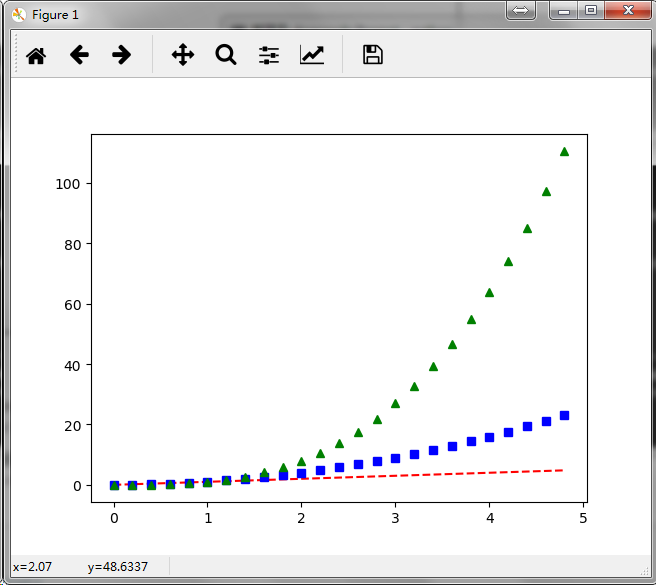补充：在 plot()  中，格式化字符串由颜色、标识符和线型三部分组成，定义格式如下：
fmt = '[color][marker][line]'
其中，支持的颜色缩写有如下几个：
charactercolor'b'blue'g'green'r'red'c'cyan'm'magenta'y'yellow'k'black'w'white
标识符定义如下表所示：
characterdescription'.'point marker','pixel marker'o'circle marker'v'triangle_down marker'^'triangle_up marker'<'triangle_left marker'>'triangle_right marker'1'tri_down marker'2'tri_up marker'3'tri_left marker'4'tri_right marker's'square marker'p'pentagon marker'*'star marker'h'hexagon1 marker'H'hexagon2 marker'+'plus marker'x'x marker'D'diamond marker'd'thin_diamond marker'|'vline marker'_'hline marker
可以选用的线型有以下四个：
characterdescription'-'solid line style'--'dashed line style'-.'dash-dot line style':'dotted line style
以下是几个格式化字符串的示例：
'b'    # blue markers with default shape
'ro'   # red circles
'g-'   # green solid line
'--'   # dashed line with default color
'k^:'  # black triangle_up markers connected by a dotted line
使用关键字绘图
Matplotlib 允许用户提供带有关键字的数据对象作为参数，一旦提供了带有关键字的数据，就能够直接使用关键字来代替这些变量去生成图形。            我们可以使用某些命令来获取到这种格式的数据，例如：numpy.recarray 和 pandas.DataFrame  。
data = {'a': np.arange(50),
'c': np.random.randint(0, 50, 50),
'd': np.random.randn(50)}
data['b'] = data['a'] + 10 * np.random.randn(50)
data['d'] = np.abs(data['d']) * 100

plt.scatter('a', 'b', c='c', s='d', data=data)
plt.xlabel('entry a')
plt.ylabel('entry b')
plt.show()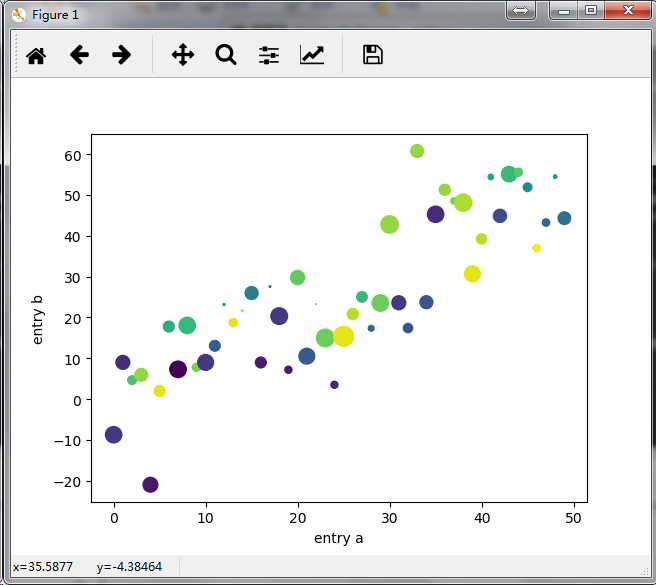使用分类变量绘图
使用分类变量来创建图形也是可以的，Matplotlib 允许用户直接把分类变量传递给多个绘图函数，例如：
names = ['group_a', 'group_b', 'group_c']
values = [1, 10, 100]

plt.figure(1, figsize=(9, 3))

plt.subplot(131)
plt.bar(names, values)
plt.subplot(132)
plt.scatter(names, values)
plt.subplot(133)
plt.plot(names, values)
plt.suptitle('Categorical Plotting')
plt.show()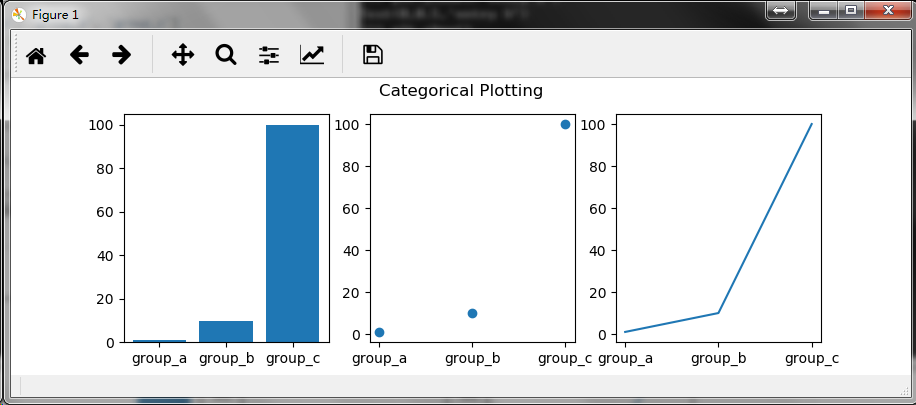控制线的属性
线的属性有很多，详情参见  matplotlib.lines.Line2D ，例如你可以设置：线宽、虚线和抗锯齿效果，等等。            我们可以通过以下几种途径对线的属性进行设置：            使用关键字参数
plt.plot(x, y, linewidth=2.0)
使用2D 线实例的set方法            plot() 命令会返回一个线对象的列表，例如：line1, line2 = plot(x1, y1, x2, y2)。在下面的代码中，我们将假设我们只有一条线即plot() 命令返回的线列表的长度为 1 。我们使用 line, 来获取列表中的第一个元素。
line, = plt.plot(x, y, '-')
line.set_antialiased(False) # turn off antialising
使用setp()命令            下面示例一下如何使用MATLAB风格的命令对一个线列表的多个属性进行设置。很明显地，setp() 能够作用于一个对象列表或者是一个单独的对象。你既可以使用Python 的关键字作为参数也可以使用MATLAB风格的string/value 对作为参数：
lines = plt.plot(x1, y1, x2, y2)
# use keyword args
plt.setp(lines, color='r', linewidth=2.0)
# or MATLAB style string value pairs
plt.setp(lines, 'color', 'r', 'linewidth', 2.0)
2D 线实例的可用属性见下表：
PropertyValue Typealphafloatanimated[True | False]antialiased or aa[True | False]clip_boxa matplotlib.transform.Bbox instanceclip_on[True | False]clip_patha Path instance and a Transform instance, a Patchcolor or cany matplotlib colorcontainsthe hit testing functiondash_capstyle['butt' | 'round' | 'projecting']dash_joinstyle['miter' | 'round' | 'bevel']dashessequence of on/off ink in pointsdata(np.array xdata, np.array ydata)figurea matplotlib.figure.Figure instancelabelany stringlinestyle or ls[ '-' | '--' | '-.' | ':' | 'steps' | ...]linewidth or lwfloat value in pointslod[True | False]marker[ '+' | ',' | '.' | '1' | '2' | '3' | '4' ]markeredgecolor or mecany matplotlib colormarkeredgewidth or mewfloat value in pointsmarkerfacecolor or mfcany matplotlib colormarkersize or msfloatmarkevery[ None | integer | (startind, stride) ]pickerused in interactive line selectionpickradiusthe line pick selection radiussolid_capstyle['butt' | 'round' | 'projecting']solid_joinstyle['miter' | 'round' | 'bevel']transforma matplotlib.transforms.Transform instancevisible[True | False]xdatanp.arrayydatanp.arrayzorderany number
如果你想要获取可供设置的线属性的列表，你可以直接调用 setp() 命令并传入一条线或者传入一个线列表作为唯一参数。
lines = plt.plot([1, 2, 3])

plt.setp(lines)
alpha: float
animated: [True | False]
antialiased or aa: [True | False]
...snip
使用多图形和多轴
MATLAB和 pyplot 都有当前图形和当前轴的概念，所有的绘图命令都会被应用到当前轴上。在 Matplotlib 中，gca() 命令用来获取当前轴（一个 matplotlib.axes.Axes 实例），gcf() 命令用来获取当前图形（一个 matplotlib.figure.Figure 实例）。通常情况下，你并不需要考虑这些，因为 Matplotlib 会在后台对它们进行自动处理。            下面是一个创建了两个子图形的示例脚本：
def f(t):
return np.exp(-t) * np.cos(2*np.pi*t)

t1 = np.arange(0.0, 5.0, 0.1)
t2 = np.arange(0.0, 5.0, 0.02)

plt.figure(1)
plt.subplot(211)
plt.plot(t1, f(t1), 'bo', t2, f(t2), 'k')

plt.subplot(212)
plt.plot(t2, np.cos(2*np.pi*t2), 'r--')
plt.show()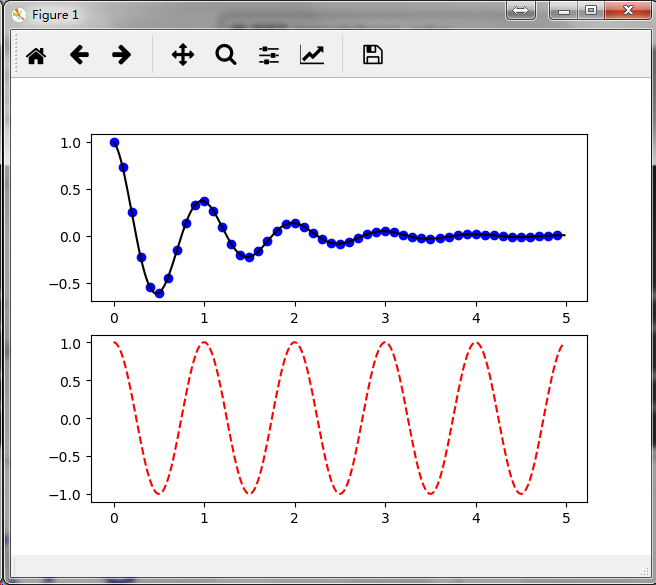figure()命令在这儿是一个可选项，因为默认创建的就是 figure(1) ，同样地，在没有手动地指定任何轴的情况下，将默认创建 subplot(111) 。subplot() 命令需要指定行数、列数、图形编号，其中图形编号的取值范围为（1 ~ 行数*列数）。如果行数*列数<10，参数之间的逗号可以省略。因此，subplot(211) 和 subplot(2, 1, 1) 的作用是一样的。
你可以创建任意数量的子图形或者坐标系。如果你想要手动去放置一个坐标系可以使用 axes() 命令，并通过  axes([left, bottom, width, height]) 的四个参数（ 0 ~ 1 的小数）来指定坐标系的位置和大小。
手动设置坐标系示例：https://matplotlib.org/gallery/subplots_axes_and_figures/axes_demo.html            绘制多个子图形示例：            https://matplotlib.org/gallery/subplots_axes_and_figures/subplot_demo.html            https://matplotlib.org/gallery/subplots_axes_and_figures/subplots_demo.html            你可以通过多次调用figure() 方法并传入一个递增的图形编号来创建多个图形。当然，只要你喜欢每一个图形也可以包含多个子图形或者坐标系：
import matplotlib.pyplot as plt
plt.figure(1)                # the first figure
plt.subplot(211)             # the first subplot in the first figure
plt.plot([1, 2, 3])
plt.subplot(212)             # the second subplot in the first figure
plt.plot([4, 5, 6])

plt.figure(2)                # a second figure
plt.plot([4, 5, 6])          # creates a subplot(111) by default

plt.figure(1)                # figure 1 current; subplot(212) still current
plt.subplot(211)             # make subplot(211) in figure1 current
plt.title('Easy as 1, 2, 3') # subplot 211 title
plt.show()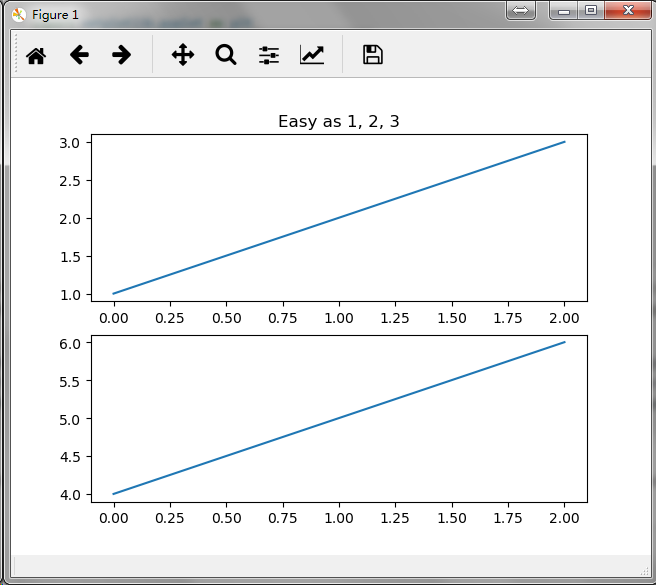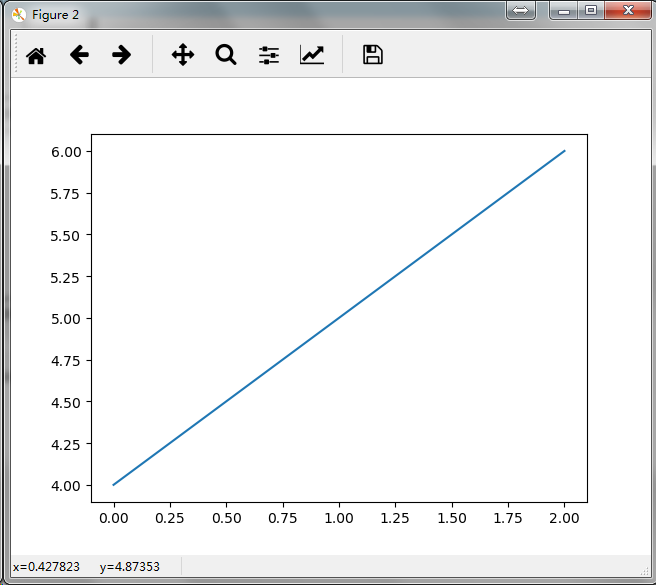你可以使用 clf() 命令来清除当前图形或者使用 cla() 命令来清除当前坐标系。 如果你觉得由后台帮你维护图形状态（指当前的图像，图形和坐标系）这种方式比较烦人，无须绝望：这只是对面向对象API的一个瘦状态封装，你可以把它替换掉（参见 Artist tutorial ）。             如果你是在创建多个图形，你还需要注意另外一件事：图形所占用的内存直到你调用 close() 显式地将其关闭才会全部释放，如果只删除掉图形的引用或者通过窗口管理器销毁掉屏幕上的图像展示窗口，图形所占用的内存是不能完全被释放的，因为pyplot 在 close() 命令被调用之前仍会对其内部引用进行维护。
使用文本
使用 text() 命令可以在图形的任意位置添加文本，xlabel()、ylabel()、title() 用来在指定位置添加文本（更多详细案例参见 Text in Matplotlib Plots ）。
mu, sigma = 100, 15
x = mu + sigma * np.random.randn(10000)

# the histogram of the data
n, bins, patches = plt.hist(x, 50, density=1, facecolor='g', alpha=0.75)

plt.xlabel('Smarts')
plt.ylabel('Probability')
plt.title('Histogram of IQ')
plt.text(60, .025, r'$\mu=100,\ \sigma=15$')
plt.axis([40, 160, 0, 0.03])
plt.grid(True)
plt.show()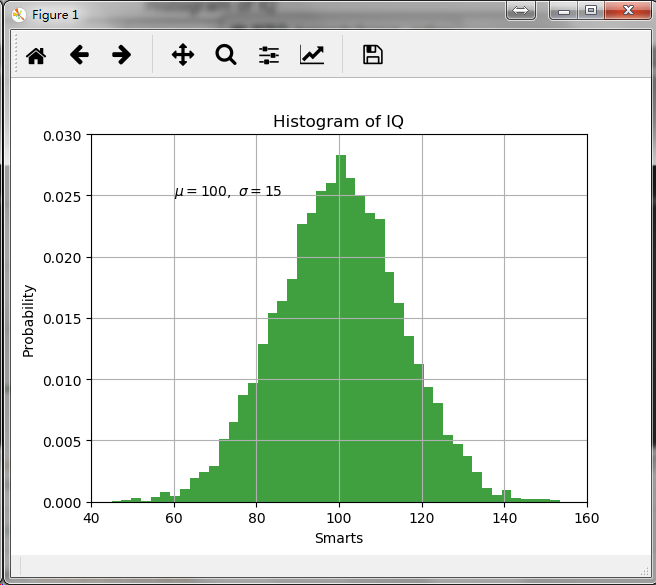所有的文本命令都会返回一个  matplotlib.text.Text  实例，跟前面线的属性设置一样，你可以往文本命令中传入关键字参数或者使用 setp() 命令来自定义文本的属性。
t = plt.xlabel('my data', fontsize=14, color='red')
关于文本的属性的更多细节请参见：https://matplotlib.org/tutorials/text/text_props.html
在文本中使用数学表达式
Matplotlib 接收的文本表达式中可以包含 TeX 方程表达式，比如，书写时，需要使用 "$" 符号把TeX表达式包起来： plt.title(r'$\sigma_i=15\$')
标题字符串前面的”r”字符非常重要：它表示这个字符串是一个原生字符串，不用把里面的反斜杠当作 Python 的转义字符来看待。            Matplotlib 有一个內建的 TeX 方程式的语法分析器和布局引擎，并带有自己的数学字体（详情参见  Writing mathematical expressions ）。因此你可以不用安装TeX就能够跨平台使用数学表达式，对于那些已经安装了LaTeX和dvipng的用户，你也可以使用LaTex 来格式化你的文字并将输出的文本并入到你要展示的图形或者要保存的脚本中（详情参见 Text rendering With LaTeX）。
注释文本
上面讲到过 text()命令的基本作用是在坐标系的任意位置添加一个文本，而文本通常是用来对图形的某些特征进行注释，annotate() 方法提供了一些非常有用的功能使得注释变得更加容易。对于注释有两个需要考虑的点：使用 xy 参数来代表需要被注释的位置；使用 xytext 参数来指定注释文本的放置位置；这两个参数的取值均为(x,y) 的组合。
ax = plt.subplot(111)

t = np.arange(0.0, 5.0, 0.01)
s = np.cos(2*np.pi*t)
line, = plt.plot(t, s, lw=2)

plt.annotate('local max', xy=(2, 1), xytext=(3, 1.5),
arrowprops=dict(facecolor='black', shrink=0.05),
)

plt.ylim(-2, 2)
plt.show()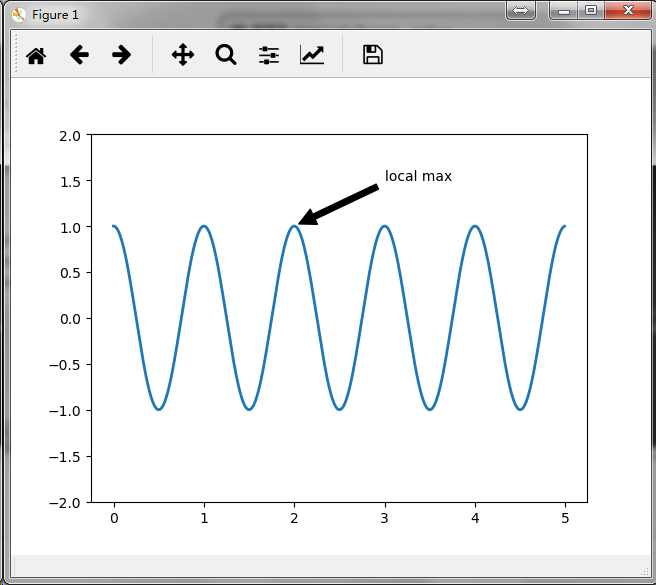在这个基础示例中，xy (箭头提示) 和 xytext(文本位置)都是在数据的坐标系中，这里还有许多其他种类的坐标系可以选择（详情参见  Basic annotation 和 Advanced Annotation），更多示例参见： Annotating Plots。
对数及其他非线性坐标系
matplotlib.pylot 除了支持线性坐标系外，也支持对数和分对数等非线性坐标系，当数据的量级跨度比较大时会经常用到这些坐标系，改变一个坐标轴的刻度非常容易：
plt.xscale('log')
下例中展示了对于同样的数据，当Y轴使用不同刻度时的四个图形：
from matplotlib.ticker import NullFormatter  # useful for logit scale

# Fixing random state for reproducibility
np.random.seed(19680801)

# make up some data in the interval ]0, 1[
y = np.random.normal(loc=0.5, scale=0.4, size=1000)
y = y[(y > 0) & (y < 1)]
y.sort()
x = np.arange(len(y))

# plot with various axes scales
plt.figure(1)

# linear
plt.subplot(221)
plt.plot(x, y)
plt.yscale('linear')
plt.title('linear')
plt.grid(True)

# log
plt.subplot(222)
plt.plot(x, y)
plt.yscale('log')
plt.title('log')
plt.grid(True)

# symmetric log
plt.subplot(223)
plt.plot(x, y - y.mean())
plt.yscale('symlog', linthreshy=0.01)
plt.title('symlog')
plt.grid(True)

# logit
plt.subplot(224)
plt.plot(x, y)
plt.yscale('logit')
plt.title('logit')
plt.grid(True)
# Format the minor tick labels of the y-axis into empty strings with
# NullFormatter, to avoid cumbering the axis with too many labels.
plt.gca().yaxis.set_minor_formatter(NullFormatter())
# Adjust the subplot layout, because the logit one may take more space
# than usual, due to y-tick labels like "1 - 10^{-3}"
wspace=0.35)

plt.show()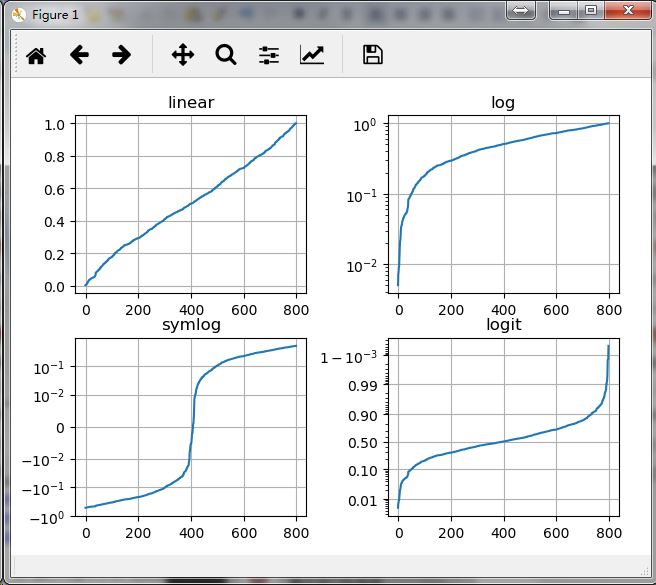你也可以添加你自定义的刻度，详情参见：Developer's guide for creating scales and transformations
展开全文• import matplotlib.pyplot as plt # 代码中的“...”代表省略的其他参数 ax = plt.subplot(111) # 设置刻度字体大小 plt.xticks(fontsize=20) plt.yticks(fontsize=20) # 设置坐标标签字体大小 ax.set_xlabel(..., ...
Background
调整字体的大小
Methods
import matplotlib.pyplot as plt

ax = plt.subplot(111)
# 设置刻度字体大小
plt.xticks(fontsize=20)
plt.yticks(fontsize=20)
# 设置坐标标签字体大小

ax.xlabel(..., fontsize=20) # 代码中的“...”代表省略的其他参数
ax.ylabel(..., fontsize=20)# 代码中的“...”代表省略的其他参数
# 设置图例字体大小
ax.legend(..., fontsize=20)# 代码中的“...”代表省略的其他参数


Result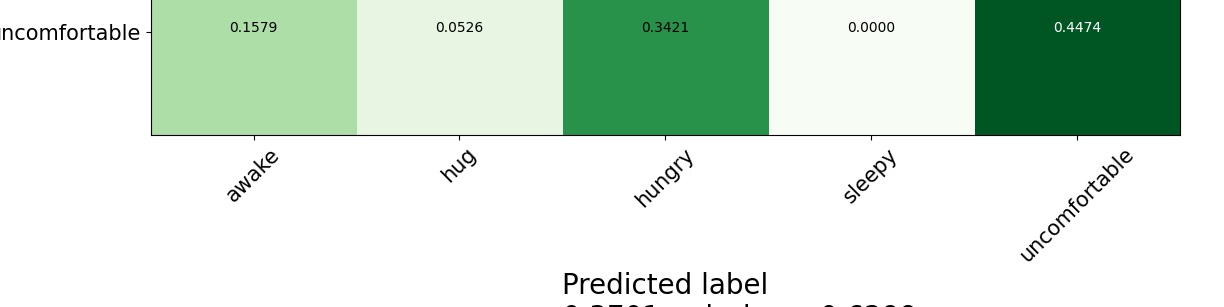展开全文plt调整字体大小
• 我相信，看到这篇博客的人，你肯定已经会使用Matplotlib中的pyplot画图。 比如下面这种图 你也应该会调整单个图的大小了，就是使用如下语句控制单个图形figure的大小，比如我这里设的8*6的。 fig3 = plt....
前段时间就遇到了这个问题，一直忘了写，今晚夜深人静总结一波~

问题

我相信，看到这篇博客的人，你肯定已经会使用Matplotlib中的pyplot画图。
比如下面这种图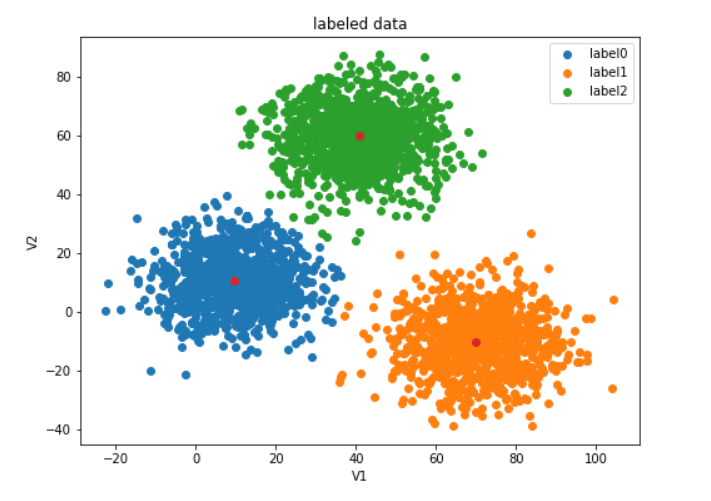你也应该会调整单个图的大小了，就是使用如下语句控制单个图形figure的大小，比如我这里设的8*6的。
fig3 = plt.figure(figsize=(8,6))
但随着继续深入的学习，有时我们很有必要将两个图画在一起，来做对比，所以你也应该会在一个画布上画多个子图了。比如下图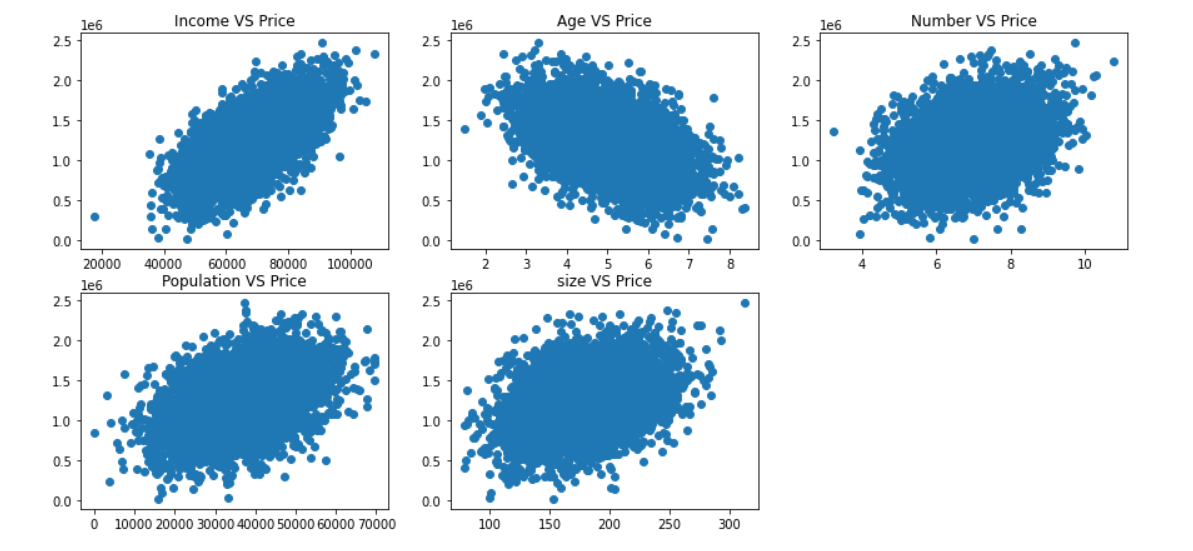即是通过subplot实现
#展示一下数据
fig = plt.figure(figsize=(15,7))

fig1 = plt.subplot(231)
plt.scatter(data.loc[:,'Avg. Area Income'],data.loc[:,'Price'])
plt.title('Income VS Price')

fig2 = plt.subplot(232)
plt.scatter(data.loc[:,'Avg. Area House Age'],data.loc[:,'Price'])
plt.title('Age VS Price')

fig3 = plt.subplot(233)
plt.scatter(data.loc[:,'Avg. Area Number of Rooms'],data.loc[:,'Price'])
plt.title('Number VS Price')

fig4 = plt.subplot(234)
plt.scatter(data.loc[:,'Area Population'],data.loc[:,'Price'])
plt.title('Population VS Price')

fig5 = plt.subplot(235)
plt.scatter(data.loc[:,'size'],data.loc[:,'Price'])
plt.title('size VS Price')
plt.show()
目前为止图好像没有问题，那问题在哪呢？就是在子图比较少的时候，整个图可能会变形，出现下图情况。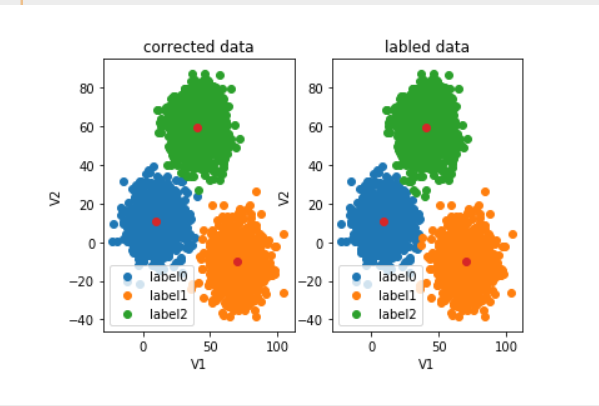这显然不是我们期望的，我们希望他不要拉长。但是貌似直接通过subplot画出的子图无法更改大小，网上给的一些方案也比较麻烦。

简便的解决方法

把这两个子图画在同一个画布里，这样即是子图无法改变，但是外面的画布大小可以改变，子图就可以根据外面画布大小自适应的显示了。
对于该图其原始代码为
fig6 = plt.subplot(121)
label0 = plt.scatter(X.loc[:,'V1'][y_corrected==0],X.loc[:,'V2'][y_corrected==0])
label1 = plt.scatter(X.loc[:,'V1'][y_corrected==1],X.loc[:,'V2'][y_corrected==1])
label2 = plt.scatter(X.loc[:,'V1'][y_corrected==2],X.loc[:,'V2'][y_corrected==2])

plt.title("corrected data")
plt.xlabel('V1')
plt.ylabel('V2')
plt.legend((label0,label1,label2),('label0','label1','label2'))
plt.scatter(centers[:,0],centers[:,1])

fig7 = plt.subplot(122)
label0 = plt.scatter(X.loc[:,'V1'][y==0],X.loc[:,'V2'][y==0])
label1 = plt.scatter(X.loc[:,'V1'][y==1],X.loc[:,'V2'][y==1])
label2 = plt.scatter(X.loc[:,'V1'][y==2],X.loc[:,'V2'][y==2])

plt.title("labled data")
plt.xlabel('V1')
plt.ylabel('V2')
plt.legend((label0,label1,label2),('label0','label1','label2'))
plt.scatter(centers[:,0],centers[:,1])
plt.show()
可以看到两个子图fig6和fig7都是直接使用subplot得到的，所以它变形了。
修改后应该是这样的：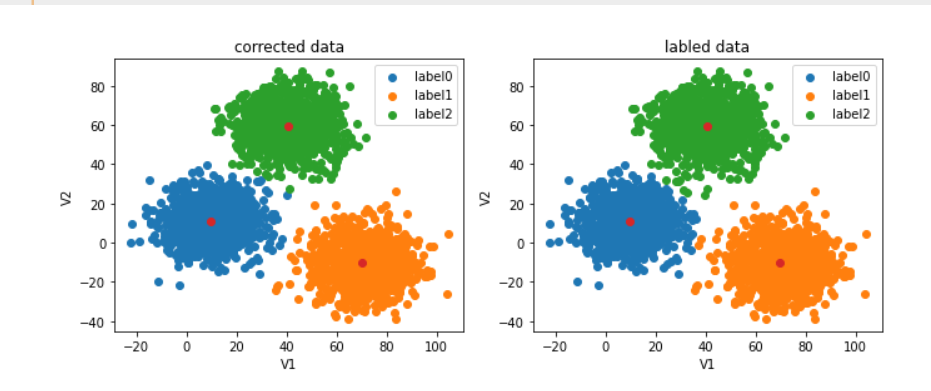对应代码 ：
fig = plt.figure(figsize=(11,4))
fig6 = plt.subplot(121)
label0 = plt.scatter(X.loc[:,'V1'][y_corrected==0],X.loc[:,'V2'][y_corrected==0])
label1 = plt.scatter(X.loc[:,'V1'][y_corrected==1],X.loc[:,'V2'][y_corrected==1])
label2 = plt.scatter(X.loc[:,'V1'][y_corrected==2],X.loc[:,'V2'][y_corrected==2])

plt.title("corrected data")
plt.xlabel('V1')
plt.ylabel('V2')
plt.legend((label0,label1,label2),('label0','label1','label2'))
plt.scatter(centers[:,0],centers[:,1])

fig7 = plt.subplot(122)
label0 = plt.scatter(X.loc[:,'V1'][y==0],X.loc[:,'V2'][y==0])
label1 = plt.scatter(X.loc[:,'V1'][y==1],X.loc[:,'V2'][y==1])
label2 = plt.scatter(X.loc[:,'V1'][y==2],X.loc[:,'V2'][y==2])

plt.title("labled data")
plt.xlabel('V1')
plt.ylabel('V2')
plt.legend((label0,label1,label2),('label0','label1','label2'))
plt.scatter(centers[:,0],centers[:,1])
plt.show()
相比原来的代码就多了第一行的操作，定一个合适画布的大小就可以方便动态调整子图了。

麻烦点的方法

看到网上是有可以自定义子图大小的方法的，不过相比我想出来的这个方法，感觉太麻烦了。这个方法能解决我这一类问题了，如果后面遇到需要一个子图大一个子图小的问题再单独记录把。
就到这把，睡觉~


展开全文python
• 本文代码运行在jupyter上 一.安装matplotlib pip3 install matplotlib 二.进入matplotlib查看源码 渐变柱状图官方示例
本文代码运行在jupyter上 一.安装matplotlib
pip3 install matplotlib
二.进入matplotlib查看源码 渐变柱状图官方示例 三.源码
import matplotlib.pyplot as plt
import numpy as np

np.random.seed(19680801)
def gradient_image(ax, extent, direction=0.3, cmap_range=(0, 1), **kwargs):
"""
Draw a gradient image based on a colormap.

Parameters
----------
ax : Axes
The axes to draw on.
extent
The extent of the image as (xmin, xmax, ymin, ymax).
By default, this is in Axes coordinates but may be
changed using the *transform* kwarg.
direction : float
The direction of the gradient. This is a number in
range 0 (=vertical) to 1 (=horizontal).
cmap_range : float, float
The fraction (cmin, cmax) of the colormap that should be
used for the gradient, where the complete colormap is (0, 1).
**kwargs
Other parameters are passed on to .Axes.imshow().
In particular useful is *cmap*.
"""
phi = direction * np.pi / 2
v = np.array([np.cos(phi), np.sin(phi)])
X = np.array([[v @ [1, 0], v @ [1, 1]],
[v @ [0, 0], v @ [0, 1]]])
a, b = cmap_range
X = a + (b - a) / X.max() * X
im = ax.imshow(X, extent=extent, interpolation='bicubic',
vmin=0, vmax=1, **kwargs)
return im
def gradient_bar(ax, x, y, width=0.5, bottom=0):
for left, top in zip(x, y):
right = left + width
cmap=plt.cm.Blues_r, cmap_range=(0, 0.8))

xmin, xmax = xlim = 0, 10
ymin, ymax = ylim = 0, 1

fig, ax = plt.subplots()
ax.set(xlim=xlim, ylim=ylim, autoscale_on=False)

# background image
gradient_image(ax, direction=0, extent=(0, 1, 0, 1), transform=ax.transAxes,
cmap=plt.cm.Oranges, cmap_range=(0.1, 0.6))

N = 10
x = np.arange(N) + 0.15
y = np.random.rand(N)
ax.set_aspect('auto')
plt.show()

四.分析
np.random.seed(19680801)
#numpy随机数，固定seed种子，这样每次生成的随机数都是固定的
def gradient_image(ax, extent, direction=0.3, cmap_range=(0, 1), **kwargs):
#定义了一个生成图片的方法，图片是渐变的
"""
ax:画板
extent:调整宽高比例
direction:颜色渐变方向（0，1）0为竖直，1为水平
cmap_range:颜色渐变的范围
**kwargs:imshow中的参数
"""
phi = direction * np.pi / 2     #角度值
v = np.array([np.cos(phi), np.sin(phi)])#求解余弦正弦
X = np.array([[v @ [1, 0], v @ [1, 1]],
[v @ [0, 0], v @ [0, 1]]])
#@号代表的是矩阵运算
a, b = cmap_range#代表着颜色的最小啊范围和最大范围
X = a + (b - a) / X.max() * X#用颜色范围调整数值
im = ax.imshow(X, extent=extent, interpolation='bicubic',
vmin=0, vmax=1, **kwargs)
"""
显示图片
vmin，vmax:图片的最大值和最小值，jpg（0，255）png（0，1）
x:显示的图片数据
interpolation:填充效果 “bicubic”渐变
"""

return im

def gradient_bar(ax, x, y, width=0.5, bottom=0):
#bottom 初始坐标零点位置
for left, top in zip(x, y):  #zip  同时取多个数组中的数据
right = left + width
cmap=plt.cm.Blues_r, cmap_range=(0, 0.8))
#cmap 柱子颜色 cmap_range 柱子颜色渐变范围
N = 10
x = np.arange(N) + 0.15  #柱子x坐标 整体右挪0.15
y = np.random.rand(N)    #柱子高度随机数
ax.set_aspect('auto')#equal设置横纵坐标显示比例 equal刻度相等，auto刻度不相等
plt.show()
展开全文python numpy
• matplotlib 柱状图之渐变色设置 matplotlib中，在用bar或者barh绘制柱状图时，发现加入cmap是不管用的，不支持这个关键字，而且网上找了许久，也没有发现有类似功能，因此，干脆自己写一个试试，说来就来！！ ...
• 使用matplotlib模块中的LinearSegmentedColormap去定义 构建一个matrix，第一列是0~1的递增列，可以是小数，将颜色分几个部分 例如：如果有五种颜色可以选择写0，0.25，0.5，0.75，1 第二列写十六进制颜色的编码即可...python
• plt.legend(prop={'family':'SimHei','size':4}) 直接这样在size里面改就可以了，不需要更新字体库什么的。码住！python 可视化
• 前面学习了怎么样找到区域，也...那么你就会好奇地问，matplotlib里怎么样设置坐标轴的刻度呢？带着这个问题，下面通过例子演示： 在这里可以看到，坐标系的原点是在（-10，-10），最大值分别是10。要设置这样的坐标python opencv
• matplotlib画图像变色问题 在使用opencv配合jupyter notebook调试，其中常常使用matplotlib来显示图像 plt.subplot(),plt.imshow(image),plt.title('Input') plt.show() 但是在实际使用过程中，我们会发现plt....
• 15) #x轴开始和结束位置 bins2 = np.linspace(0, 9, 4) #横坐标起始和结束值，分割成 4份， plt.yticks(bins2) #设置x轴 plt.ylim(0, 9) #x轴开始和结束位置 plt.show() (80条消息)matplotlib (mplot3d) - 怎样拉伸...
• Talk is cheap, show me the code. ...import matplotlib.pyplot as plt import numpy as np x = np.arange(0, 2 * np.pi, 0.01) y = np.sin(x) plt.figure(figsize=(2,3), dpi=300) plt.plot(x,y) plt.show() 在...
• matplotlib如何设置点的像素大小，具体情况如下图。 解决方法： 将plot方法更换为scatter方法，然后就可以指定s（像素属性）的值了。 方法说明： plt.scatter(x, y, s=None, c=None, marker=None, cmap=None, norm=...python
• 终于到了python数据分析三剑客的最后一课：Matplotlib，一个专门用于绘制图形的库，话不多说，直接进入主题。内容目录图形的构成plot样式风格plot保存图像各种图形绘制1 图形的构成1.1 Figure在绘制图形之前，我们...
• matplotlib.pyplot.figure 函数内设置，参数名称为figsize。 匹配的关闭函数为matplotlib.pyplot.close 详情参考：...python 作图
• 平均人气.values) plt.ylabel('金主数量') bar_data.head() 参考文章： https://blog.csdn.net/xiaoyw71/article/details/108845807 Python Matplotlib绘制渐变色柱状图（bar）并加边框和配置渐变颜色条（colorbar）...
• 导语Seaborn和Matplotlib是Python最强大的两个可视化库。Seaborn其默认主题让人惊讶，而Matplotlib可以通过其多个分类为用户打造专属功能。01导入包102参数介绍Figure：面板(图)，matplotlib中的所有图像都是位于...
• Matplotlib使简单的事情得容易，而困难的事情得可能。 Matplotlib附带了几个附加工具包，包括使用mplot3d进行3D绘图，axes_grid1中的axis助手和axisartist中的axis助手。 大量的第三方软件包扩展并建立在...开源软件
• matplotlib画二维图像时，默认情况下的横坐标和纵坐标显示的值有时达不到自己的需求，需要借助xticks()和yticks()分别对横坐标x-axis和纵坐标y-axis进行设置。 import numpy as np import matplotlib.pyplot as ...
• matplotlib.pyplot是一些命令行风格函数的集合，使matplotlib以类似于MATLAB的方式工作。每个pyplot函数对一幅图片(figure)做一些改动：比如创建新图片，在图片创建一个新的作图区域(plotting area)，在一个作图区域...
• import matplotlib.pyplot as plt # 1、图形绘制 x = np.linspace(0,2*np.pi) # x轴 # y轴 y = np.sin(x) # 正弦 # 绘制线形图 # 调整尺⼨ plt.figure(figsize=(9,6)) plt.plot(x,y) # 2、图例 plt.plot(x,np.cos...数据可视化
• plt.figure要写在plt.plot之前才会有大小的效果python
• 本文实例为大家分享了python3使用matplotlib绘制条形图的具体代码，供大家参考，具体内容如下 绘制竖状条形图 代码 from matplotlib import pyplot as plt from matplotlib import font_manager my_font = font_...
• from matplotlib import pyplot as plt x = range(1,10) #x轴的位置 y = [6,7,12,12,15,17,15,20,18] #y轴的位置 #传入x,y，通过plot画图,并设置折线颜色、透明度、折线样式和折线宽度 标记点、标记点大小、标记点边...
• matplotlib绘图】调整图片尺寸大小 核心代码如下： import matplotlib.pyplot as plt plt.rcParams['figure.figsize'] = (12.0, 8.0) # 单位是inches 应用示例 import numpy as np import matplotlib as mpl ...python绘图 可视化 数据可视化
• 我们会说明如何设置图中：曲线拐点形状的大小，横纵坐标刻度、标签的大小、图例中形状的大小 ...import matplotlib.pyplot as plt from pylab import * FontSize=15 x=range(0,24) #下面四句对应图中四条线的纵...
• 赛璐Easy Easy Matplotlib动画创建动画应该很容易。 通过该模块，可以轻松调整现有的可视化代码来创建动画。 安装pip安装赛璐oid赛璐oid Easy Matplotlib动画创建动画应该很容易。 通过该模块，可以轻松调整现有的......